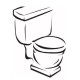# Simplifying a sum of consecutive cubes

If you were asked to find the sum of the first four cubes, you would easily be able to do so:$1^3 + 2^3 + 3^3 + 4^3 = 100$

But here you may notice something interesting:$1^3 + 2^3 + 3^3 + 4^3 = (1+2+3+4)^2$

It turns out that this holds for the sum of the first$n$ cubes for any$n$ — an identity. This identity can be written also as:$\displaystyle \sum_{i=1}^n i^3 = \left( \displaystyle \sum_{i=1}^n i \right)^2$

A second way of expressing the same identity simplifies the right side and expresses it as a combination:$\displaystyle \sum_{i=1}^n i^3 = \binom{n+1}{2}^2$

### A proof by bijection

This identity can be proven combinatorially by noticing that$\binom{n+1}{2}$ is the number of ways to choose 2 elements with repetition from the set {1..n} — the following is a proof by Benjamin and Orrison.

In this proof, we prove the third form of the identity$\displaystyle \sum_{i=1}^n i^3 = \binom{n+1}{2}^2$

by constructing one set of size$\displaystyle \sum_{i=1}^n i^3$ and constructing another set of size$\binom{n+1}{2}^2$, then constructing a bijection between the two sets.

Let$S$ be the set of ordered 4-pairs$(a,b,c,d)$ such that$1 \leq a,b,c \leq d \leq n$ — that is, the fourth integer of the pair is greater or equal to each of the other three. If we fix$d$, the number of possibilities for the variables$a,b,c$ is$d^3$. As$d$ ranges from 1 to n, the total number of elements in$S$ is equal to$\displaystyle \sum_{i=1}^n i^3$.

Also, since$\binom{n+1}{2}$ is the number of ways to choose 2 elements with repetition from the set {1..n}, there are$\binom{n+1}{2}$ pairs of integers$(h,i)$ satisfying$1 \leq h \leq i \leq n$. So let$T$ be the set of pairs of such pairs$((h,i),(j,k))$ where$1 \leq h \leq i \leq n$ and$1 \leq j \leq k \leq n$. The number of elements in$T$ is then$\binom{n+1}{2}^2$.

We further partition the sets as follows: let$S_1$ be the subset of$S$ where$a \leq b$ and let$S_2$ be the subset of$S$ where$a > b$. Similarly, let$T_1$ be the subset of$T$ where$i \leq k$ and let$T_2$ be the subset of$T$ where$i > k$. We can construct a bijection between$S$ and$T$ by constructing two bijections:$S_1 \leftrightarrow T_1$$S_2 \leftrightarrow T_2$

The following is trivially a bijection from$S_1$ to$T_1$ — the case where$a \leq b$:$(a,b,c,d) \rightarrow ((a,b),(c,d))$

The equivalent bijection from$S_2$ to$T_2$ — the case where$a > b$:$(a,b,c,d) \rightarrow ((c,d),(b,a-1))$

We can confirm that the two operations we defined are indeed bijections. This proves the identity.

## 3 thoughts on “Simplifying a sum of consecutive cubes”

1.JL says:

Would it not be easier to inductively prove that the sum of the first n integers is n(n+1)/2 and that the sum of the first n cubes is n^2(n+1)^2/4, which equals (n(n+1)/2)^2?

Like

1.luckytoilet says:

Shhhh 😛
Besides, any bijective proof beats any boring inductive argument xD

Like

2.Anonymous says:

Bijections are awesome 😛